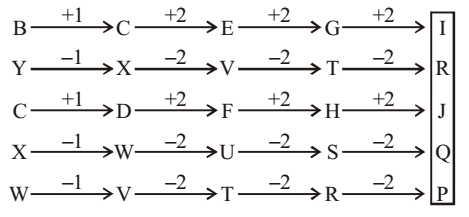## Number Series

#### Number Series

Direction: Read the given series and complete this series.

1. BYCXW, CXDWV, EVFUT, GTHSR, ?
1. IRJQP
2. KPOLN
3. KPLON
4. JOKPO
5. None of these

1. From given figure , we can see that
The next term of above given series is IRJQP .

##### Correct Option: A

From given figure , it is clear that
On adding 2 and subtracting 2 alternately in fourth term of given series .
We get , The next term of above given series is IRJQP .1. QPO, SRQ, UTS, WVU, (?)
1. XVZ
2. YXW
3. ZYA
4. VWX
5. None of these

1. From given figure , it is clear that Each letter moves +2 steps.

##### Correct Option: B

From given figure , it is clear that
On adding 2 in Each letter of given series .We get , The next term of above given series is YXW.
Hence the correct option will be B .

1. AZ, CX, FU, (?)
1. IR
2. JQ
3. IV
4. KP
5. None of these

1. The given series is in the below pattern,
1st letter moves +2, +3 and +4 steps respectively.
2nd letter moves –2, –3 and –4 steps respectively.

##### Correct Option: B

The given series is in the below pattern,
1st letter moves +2, +3 and +4 steps respectively.
2nd letter moves –2, –3 and –4 steps respectively.
Hence the place value of ? is JQ .

1. OTE, PUF, QVG, RWH, ?
1. SYJ
2. TXI
3. SXJ
4. SXI
5. None of these

1. As per the details in the question the series is in the form of
The first letters of the terms are in alphabetical order, and so are the second and third letters.

##### Correct Option: D

As per the details in the question the series is in the form of
The first letters of the terms are in alphabetical order, and so are the second and third letters.
Hence required answer will be SXI .

1. MHZ, NIW, OKT, PNQ, ?
1. RRN
2. QRN
3. QRM
4. QQN
5. None of these

1. The first letters of the terms are consecutive letters. The third letter of each term is moved three steps backward to obtain the third letter of the successive term. The middle letters of the first, second, third and fourth terms are moved one, two, three, and four steps forward respectively to obtain the middle letter of the successive terms.

##### Correct Option: B

The first letters of the terms are consecutive letters. The third letter of each term is moved three steps backward to obtain the third letter of the successive term. The middle letters of the first, second, third and fourth terms are moved one, two, three, and four steps forward respectively to obtain the middle letter of the successive terms.
The order of above series given as - + 1 , + 2 , - 3
MHZ, NIW, OKT, PNQ, ( ? )
So ? = QRN → 17 18 14
Hence , required answer will be QRN .